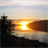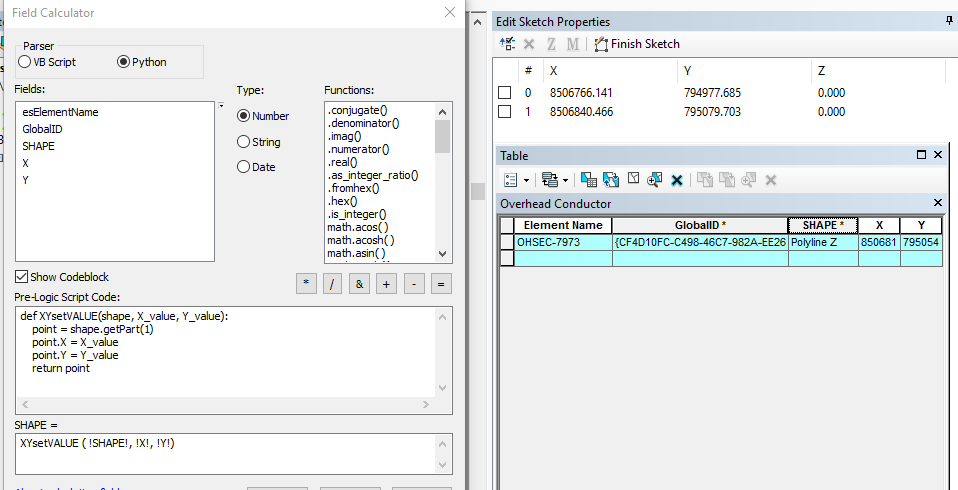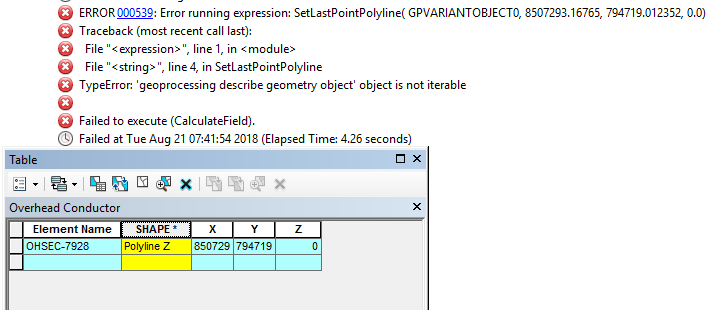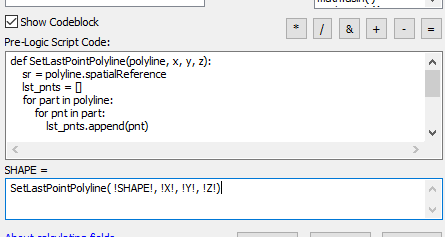Move polyline endpoints using field calculator?

516
6
08-20-2018 05:41 PMOccasional Contributor II

How do I go about moving the endpoint of a polyline using field calculator? I have the x,y end point coordinates in the "X" and "Y" fields.

def XYsetVALUE(shape, X_value, Y_value):
point = shape.getPart(1)
point.X = X_value
point.Y = Y_value
return point

SHAPE = XYsetVALUE ( !SHAPE!, !X!, !Y!)Tags (5)
1 Solution

Accepted SolutionsbyEsri Esteemed Contributor

The fact that a polyline disappears when the Z value is invalid is behavior that I have seen before (recently also with ArcGIS Pro). I assume that the geometry is considered not to be valid.

So you could follow the advise of Joshua Bixby and use the cursor. Always test this on a copy to avoid loosing data, since that data is modified.

Here is a snippet of code that you could try. It will not process multipart polylines nor elements that have an invalid Z:

def main():
import arcpy

fc = r"path to a copy of your polyline features"
fld_x = "X"
fld_y = "Y"
fld_z = "Z"

flds = ('SHAPE@', fld_x, fld_y, fld_z)
with arcpy.da.UpdateCursor(fc, flds) as curs:
for row in curs:
polyline = row
x = row
y = row
z = row
polyline = SetLastPointPolyline(polyline, x, y, z)
curs.updateRow((polyline, x, y, z, ))

def SetLastPointPolyline(polyline, x, y, z):
# BEWARE: it will not change multipart polylines or items with Z is None
if polyline.isMultipart == True:
return polyline
elif z == None:
return polyline
else:
sr = polyline.spatialReference
lst_pnts = []
for part in polyline:
for pnt in part:
lst_pnts.append(pnt)
pnt_z = arcpy.Point(x, y, z)
print lst_pnts
lst_pnts[-1] = pnt_z
print lst_pnts
polyline = arcpy.Polyline(arcpy.Array(lst_pnts), sr, True, False)
return polyline

if __name__ == '__main__':
main()

The code is not tested. If you are able to share a part of your data I can test it.

6 RepliesbyEsri Esteemed Contributor

The point is that you can read the lastPoint from a Polyline, however, it is read only, so you can't set the new value. To do this you would have to loop over the points that construct the polyline and replace the last item. It is also important that you return a valid polyline that will be written to the featureclass.

I also notice that you have the Z value enabled of your polyline geometry. This will make it a little more complicated, but I guess that you could do this with the following snippet (if the line is not a multi part):

def SetLastPointPolyline(polyline, x, y, z):
sr = polyline.spatialReference
lst_pnts = []
for part in polyline:
for pnt in part:
lst_pnts.append(pnt)
pnt_z = arcpy.Point(x, y, z)
print lst_pnts
lst_pnts[-1] = pnt_z
print lst_pnts
polyline = arcpy.Polyline(arcpy.Array(lst_pnts), sr, True, False)
return polylineOccasional Contributor II

Hi Xander,

The vast majority of my lines are not multipart, which is good. When I run it using a NULL z value the line disappears. When I set a z value it gives me the following error:Codeblock:byEsri Esteemed Contributor

The fact that a polyline disappears when the Z value is invalid is behavior that I have seen before (recently also with ArcGIS Pro). I assume that the geometry is considered not to be valid.

So you could follow the advise of Joshua Bixby and use the cursor. Always test this on a copy to avoid loosing data, since that data is modified.

Here is a snippet of code that you could try. It will not process multipart polylines nor elements that have an invalid Z:

def main():
import arcpy

fc = r"path to a copy of your polyline features"
fld_x = "X"
fld_y = "Y"
fld_z = "Z"

flds = ('SHAPE@', fld_x, fld_y, fld_z)
with arcpy.da.UpdateCursor(fc, flds) as curs:
for row in curs:
polyline = row
x = row
y = row
z = row
polyline = SetLastPointPolyline(polyline, x, y, z)
curs.updateRow((polyline, x, y, z, ))

def SetLastPointPolyline(polyline, x, y, z):
# BEWARE: it will not change multipart polylines or items with Z is None
if polyline.isMultipart == True:
return polyline
elif z == None:
return polyline
else:
sr = polyline.spatialReference
lst_pnts = []
for part in polyline:
for pnt in part:
lst_pnts.append(pnt)
pnt_z = arcpy.Point(x, y, z)
print lst_pnts
lst_pnts[-1] = pnt_z
print lst_pnts
polyline = arcpy.Polyline(arcpy.Array(lst_pnts), sr, True, False)
return polyline

if __name__ == '__main__':
main()

The code is not tested. If you are able to share a part of your data I can test it.byMVP Esteemed Contributor

elif z == None  OK

elif z is None PreferredbyMVP Esteemed Contributor

I typically use Update Cursors for such activities, I can't recall whether Field Calculator even let's someone update the geometry field.

You are calling getPart(1), are these multi-part lines?Occasional Contributor II

No, they are not multipart. I used this blog to update point locations using field calculator successfully: